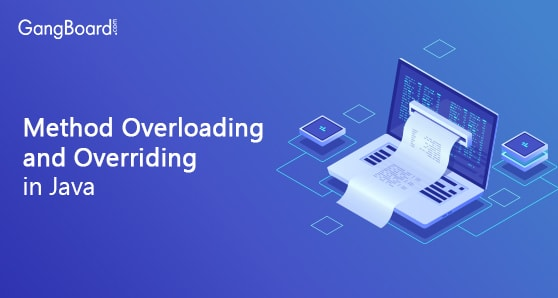Special Offer - Enroll Now and Get 2 Course at ₹25000/- Only Explore Now!May 4th, 2019

Methods having same name but the functions it performs are different from one another is method overloading in java.
Though multiple methods have same name in one class but the parameters or number of parameter and data type of parameters varies from one to another method. So in this way when we are calling the method of same name also through  parameter we can call the distinct method.

#### Example :

 ```public class Sum { // Overloaded add(). This sum takes two int parameters public int add(int x, int y) { return (x + y); } // Overloaded add(). This sum takes three int parameters public int add(int x, int y, int z) { return (x + y + z); } // Overloaded add(). This sum takes two double parameters public double add(double x, double y) { return (x + y); } // Driver code public static void main(String args[]) { Sum s = new Sum(); System.out.println(s.add(10, 20)); System.out.println(s.add(10, 20, 30)); System.out.println(s.add(10.5, 20.5)); } }```
Output :
 30 60 31.0

### Method Overriding in Java

Overriding means when a child class extends a parent class then all of it data and methods are accessible and come to the child class, but if the child class wants to  overide a method of the parent class then it can overwrite the same method in the child class. So when an object is create for the child class ,first the object checks whether the method is available in the local class , if so it take the method of the local class , if it not available in the local class then it goes and searched in the parent class.
Example :

 ```class Animal{ public void run() { System.out.println(“Animal is running"); } } class Deer extends Animal{ public void run(){ S.O.P  (“Deer is running"); } public static void main( String args[]) { Deer obj = new Deer(); obj.run(); } }```

#### Output :

 Deer is running